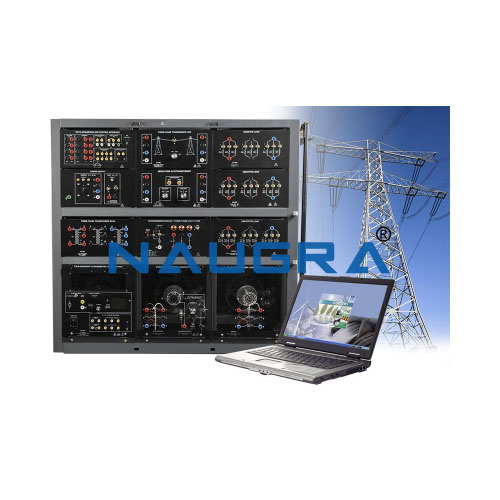# Electrical Transmission Lines Lab## Electrical Transmission Lines Lab

Lab Catalog Supply Code: NLT – 18-06-18-0021

Electrical Transmission Lines Lab
The Lab Comprising the following:
The Lab Should Cover the Following Training Contents (like or better than): Studies of three-phase lines:
•    Voltage increases on open-circuit lines
•    Voltage drops as a function of the line length
•    Voltage drops as a function of cos phi
•    Capacitive and inductive power losses on the line as a function of voltage and current
•    Phase-shift on the line
•    Control and measurement using SCADA
Study of parallel lines:
•    Power and current distribution on series and parallel lines with identical length
•    Power and current distribution on series and parallel lines with different length
•    Load distribution, power flow
•    Voltage distribution
•    Control and measurement using SCADA Study of lines with earth-fault compensation:
•    Earth fault on a spur line with an isolated neutral
•    Earth fault in meshed networks
•    Earth fault compensation
•    Control and measurement using SCADA Three phase Under Ground Cables:
•    Ferranti effect, charging capacity, critical length
•    Resistive, inductive and resistive-inductive mixed loads
•    Compensation for a resistive-inductive load
•    Determining zero impedance
•    Symmetric and asymmetric shorts
•    Dealing with star points and shorts to earth
•    Control and measurement using SCADA
Current-independent over-current time protection for lines:
•    Adjusting the parameters of the over-current-time protection
•    Determining the resetting ratio for a single-. Double- or three-pole shortcircuit
•    Control and measurement using SCADA Energy consumption measurements:
•    Three-phase loads in a star circuit (R. L or C load)
•    Three-phase loads in a delta circuit (R. L or C load)
•    Symmetrical three-phase load with RL. RC or RLC loads
Measurement with kWh and kVArh meters for symmetric & asymmetric RL loads
•    Measurement with kWh and kVArh meters during phase failure
•    Measurement with kWh and kVArh meters with overcompensation (RC load)
•    Measurement with kWh and kVArh meters for active loads
•    Measurement with kWh and kVArh meters for energy flow reversal
•    Control and measurement using SCADA

Electrical Transmission Lines Lab Operation Manual, Lab Instructor Guide and Student Workbook. Electrical Transmission Lines Lab for Educational Vocational, Didactic Technical Training Equipment Labs.